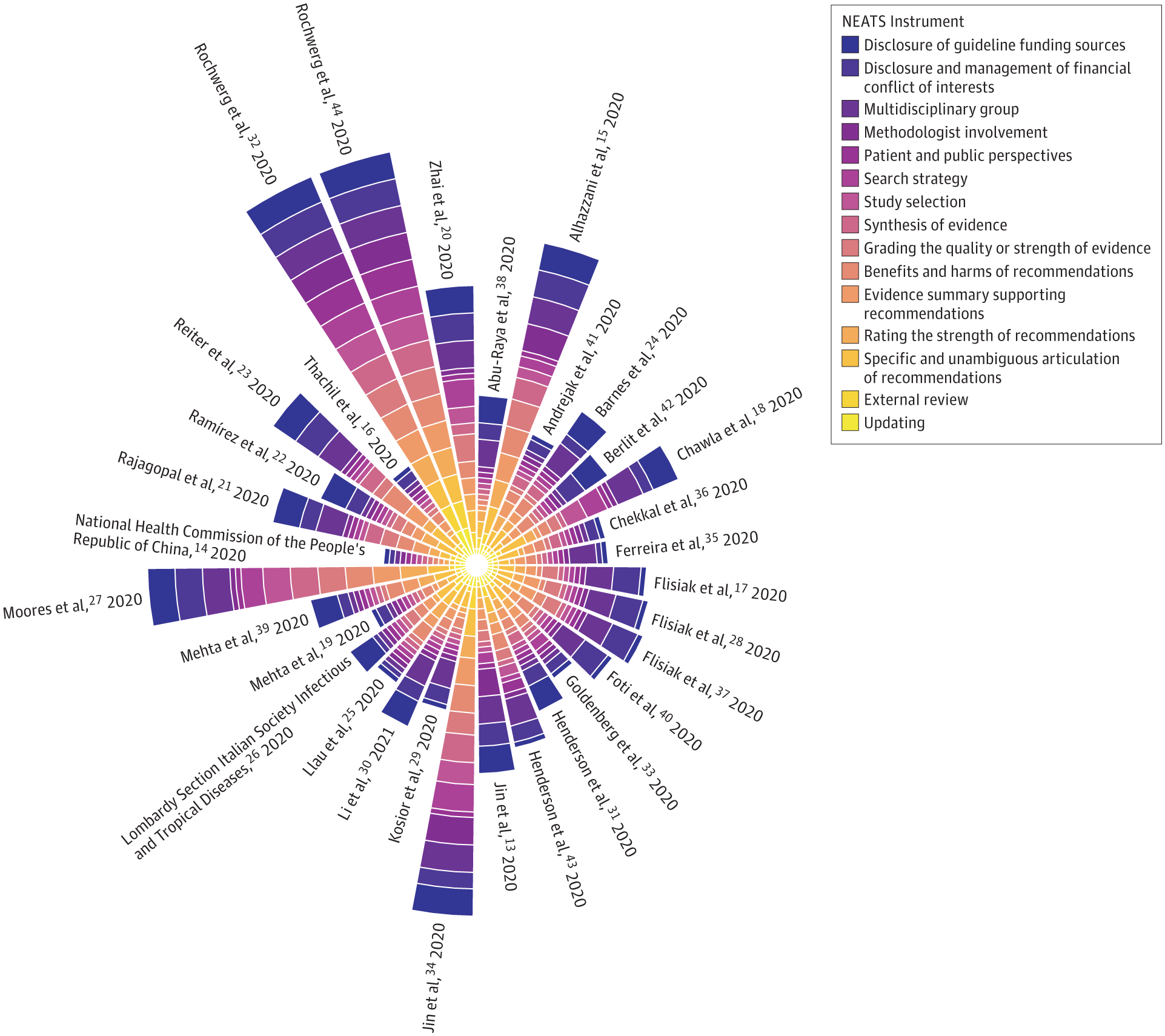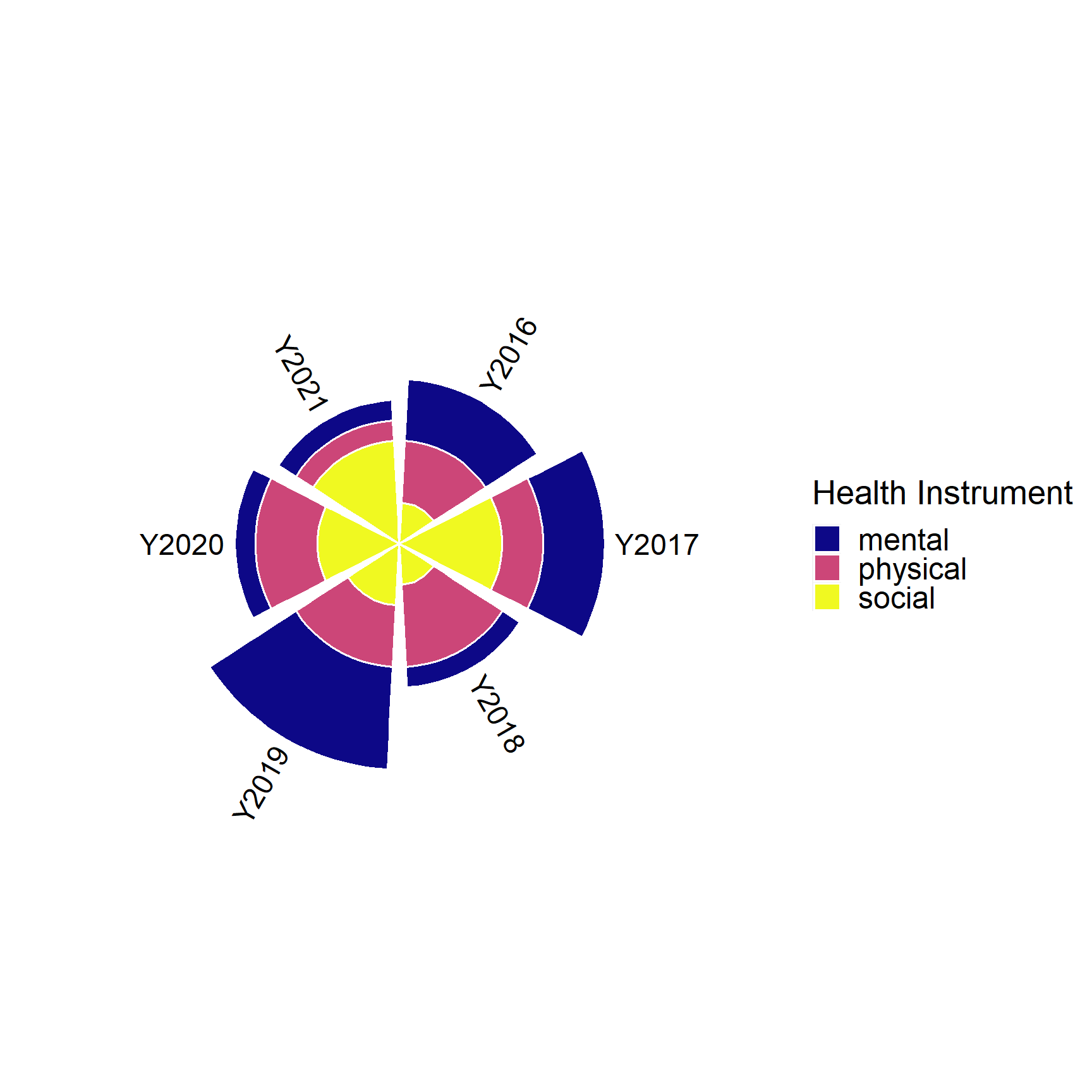Example plot from Burns et al. JAMA Network Open (2021)

## Creating Nightingale rose diagram using ggplot (static figure)

• This plot is also called Wind Rose and Coxcomb diagram.

• This diagram was developed by Florence Nightingale (1820-1910), a statistician, nurse and social reformer who was the foundational philosopher of modern nursing.

• For demonstration purpose, I will simulate a simple data inspired by the example figure.

• Suppose we have three variables in our data

• Year: 2016 to 2021
• Instruments of health: physical, mental and social
• my self-reported measure of health by instruments: a score between 1 and 5, where 5 represents the highest level of self-reported health
``````library(tidyverse)
library(DT)

set.seed(123)
dat <- tibble(
Year = paste0("Y",rep(2016:2021, each=3)),
Instrument = rep(c("physical","mental","social"),6),
Score = sample(1:5, size = 3*6, replace = T))

dat %>% datatable(
rownames = FALSE,
options = list(
columnDefs = list(list(className = 'dt-center',
targets = 0:2))))``````
• we plot year as our rays (or rose petals) and instruments as stacked bars with height representing the scores.

• Adding labels on top of each ray. This step requires we position the label using angle degrees.

``````library(viridis)

p <- ggplot(dat) +
geom_bar(aes(x=Year, y=Score, fill=Instrument), stat="identity", size=0.6, color="white") +
scale_fill_viridis(discrete=TRUE, direction = 1, option = "C", name="Health Instrument") +
ylim(0,15) + #using -1 as the lower bound to leave space in the centre of the polar
coord_polar() +
theme(
panel.background = element_rect(fill = "white", colour = "white"),
panel.grid.major = element_blank(),
panel.grid.minor = element_blank(),
panel.border = element_blank(),
axis.line = element_blank(),
axis.ticks = element_blank(),
legend.position = "right",
legend.text = element_text(size=18), #large font for website display
legend.title = element_text(size=20), #large font for website display
axis.text = element_blank(),
axis.title = element_blank())

# Add labels on top of each bar
label_data <- dat %>% group_by(Year) %>% summarize(Tot=sum(Score))
angle= round(90 - 360 * (c(1:nrow(label_data))-0.5) /nrow(label_data),0)     # I substract 0.5 because the letter must have the angle of the centre of the bars. Not extreme right(1) or extreme left (0)
label_data\$hjust<-ifelse(angle < -90, 1, 0)
label_data\$angle<-ifelse(angle < -90, angle+180, angle)

p + geom_text(data=label_data, aes(x=Year, y=Tot+0.5, label=Year, hjust=hjust),
color="black", size=6, angle= label_data\$angle, inherit.aes = FALSE)``````## Creating interactive Nightingale rose diagram using plotly

• In Plotly, r means radius and theta means angle. To specify categorical angle labels and ticks, we need to input character variable.

• Because we specified theta to be categorical (representing variable Year), we need to define even breaks between 0 to 360 by the number of unique Years (6 years in this example) to place the angle labels.

• This is controlled by setting `period = 6` under the layout option of polar.
• The interactive feature of plotly is super nice! You can try scaling and rotating the plot!

``````library(plotly)

fig <- plot_ly() %>%
data = dat,
theta = ~Year, #angle
type="barpolar",
color = ~Instrument,
hovertemplate = paste('Score: %{r}',
'<br>Year: %{theta}<br>')) %>%
layout(
legend=list(title=list(text='Health Instrument')),
polar = list(angularaxis = list(
rotation = 90,
direction = 'clockwise', #position the polar diagram
period = 6)), #evenly distribute angles to host categorical var;
margin = 0.01)
fig``````

## R session information

``````## R version 4.0.5 (2021-03-31)
## Platform: x86_64-w64-mingw32/x64 (64-bit)
## Running under: Windows 10 x64 (build 19044)
##
## Matrix products:
##
## locale: